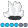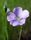## Displaying a 0 in proc freq when there is a missing level of a variable

Hello,

I am running a proc freq and I would like my output to include a level even if there are no values for it. I need this because I am using my output to generate a bar chart in Excel and for some levels there are no observations but I want that information to be shown in the chart. I am using this code to generate the output below. As you can see for GRAPHVAR there are no observations for level 2 but I would like the output to have a line in it where GRAPHVAR=2 AND FREQUENCY=0. Is this possible?

PROC FREQ DATA=TEST NLEVELS;

BY SRVC;

TABLES GRAPHVAR*COST_RANGE/LIST MISSING;

RUN;

GRAPHVAR COST_RANGE Frequency Percent Cumulative
Frequency Cumulative
Percent
 1 \$27,350 and Below 6 4.55 6 4.55 3 \$29,350-\$31,350 21 15.91 27 20.45 4 \$31,350-\$33,350 83 62.88 110 83.33 5 \$33,350-\$35,350 16 12.12 126 95.45 6 \$35,350-\$37,350 2 1.52 128 9.97 7 Above \$37,350 4 3.03 132 100

Number of Variable Levels Variable Levels
 GRAPHVAR 6 COST_RANGE 6
1 ACCEPTED SOLUTION

Accepted Solutions

## Re: Displaying a 0 in proc freq when there is a missing level of a variable

Since there is no data Proc freq has no way to add a count for , I presume, a value of 2 for your graph_var.

There are a couple of things that might work.

One would be to create a data set with the values of the categorical value you need and combine with the results of Proc Freq output;

Here is a brief skeleton of this approach, some details regarding you BY and Cost_Range variables will need to be provided by you.

``````Data full;
do SRVC = (your values needed to match the BY Group variable in your data);
do Graph_var= 1 to 7;
count=0;
output;
end;
end;
run;

PROC FREQ DATA=TEST NLEVELS;
BY SRVC;
TABLES GRAPHVAR*COST_RANGE/LIST MISSING out=FreqOut;

RUN;

data want;
update full freqout;
by SRVC Graph_var;
run;``````

AT which point I would go to a SAS procedure to create the graphs, especially with BY Group processing.

If you must export to Excel then perhaps proc print will get what you want as far as your BY, Graph_var and count variables.

5 REPLIES 5

## Re: Displaying a 0 in proc freq when there is a missing level of a variable

Since there is no data Proc freq has no way to add a count for , I presume, a value of 2 for your graph_var.

There are a couple of things that might work.

One would be to create a data set with the values of the categorical value you need and combine with the results of Proc Freq output;

Here is a brief skeleton of this approach, some details regarding you BY and Cost_Range variables will need to be provided by you.

``````Data full;
do SRVC = (your values needed to match the BY Group variable in your data);
do Graph_var= 1 to 7;
count=0;
output;
end;
end;
run;

PROC FREQ DATA=TEST NLEVELS;
BY SRVC;
TABLES GRAPHVAR*COST_RANGE/LIST MISSING out=FreqOut;

RUN;

data want;
update full freqout;
by SRVC Graph_var;
run;``````

AT which point I would go to a SAS procedure to create the graphs, especially with BY Group processing.

If you must export to Excel then perhaps proc print will get what you want as far as your BY, Graph_var and count variables.Pamela_JSRCC
Quartz | Level 8

## Re: Displaying a 0 in proc freq when there is a missing level of a variable

One way is to create a table of the possible graphvar values and use a left join with the output table from proc freq.  You loose the cumulative count and frequency, but hopefully that is not important.

data graphvar_tbl;

input graphvar cost_range \$20.;

datalines;

1 \$27,350 and Below

2 \$27,350-\$29,350

3 \$29,350-\$31,350

4 \$31,350-\$33,350

5 \$33,350-\$35,350

6 \$35,350-\$37,350

7 Above \$37,350

;

data test;

srvc = 0;

graphvar = 1; do i=1 to 6; output; end;

graphvar = 3; do i=1 to 21; output; end;

graphvar = 4; do i=1 to 83; output; end;

graphvar = 5; do i=1 to 16; output; end;

graphvar = 6; do i=1 to 2; output; end;

graphvar = 7; do i=1 to 4; output; end;

run;

PROC FREQ DATA=TEST NLEVELS;

BY srvc;

TABLES GRAPHVAR/LIST MISSING out=test_freq;

RUN;

proc contents varnum data=test_freq;

/*

# Variable  Type Len Label

1 srvc      Num  8

2 graphvar  Num  8

3 COUNT     Num  8   Frequency Count

4 PERCENT   Num  8   Percent of Total Frequency

*/

proc sql;

create table test_results as

select coalesce(b.srvc,0)               as srvc,

a.graphvar, a.cost_range,

coalesce(b.count,0)              as count,

coalesce(b.percent,0) format 5.2 as percent

from      graphvar_tbl A

left join test_freq    B on b.graphvar = a.graphvar

;

select * from test_results;

/*

srvc graphvar cost_range count percent

-----------------------------------------------------------

0 1 \$27,350 and Below 6  4.55

0 2 \$27,350-\$29,350   0  0.00

0 3 \$29,350-\$31,350  21 15.91

0 4 \$31,350-\$33,350  83 62.88

0 5 \$33,350-\$35,350  16 12.12

0 6 \$35,350-\$37,350   2  1.52

0 7 Above \$37,350     4  3.03

*/LinusH
Tourmaline | Level 20

## Re: Displaying a 0 in proc freq when there is a missing level of a variable

Perhaps this may apply to your request:

http://support.sas.com/techsup/notes/v8/25/056.html
Data never sleepsKsharp
Super User

## Re: Displaying a 0 in proc freq when there is a missing level of a variable

You can use WEIGHT statement + ZERO option.
```
data test;
set test ;
BY SRVC;
w=1;
output;
if last.SRVC then do;
w=0;
GRAPHVAR=2;
output;
end;
run;

PROC FREQ DATA=TEST NLEVELS;
BY SRVC;
TABLES GRAPHVAR*COST_RANGE/LIST MISSING;
weight w / zero ;
RUN;

```